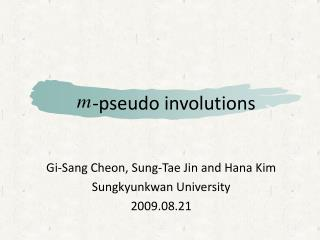DownloadDownload Presentation-pseudo involutions

# -pseudo involutions

Télécharger la présentation## -pseudo involutions

- - - - - - - - - - - - - - - - - - - - - - - - - - - E N D - - - - - - - - - - - - - - - - - - - - - - - - - - -
##### Presentation Transcript

1. -pseudo involutions Gi-Sang Cheon, Sung-Tae Jin and Hana Kim Sungkyunkwan University 2009.08.21

2. Contents • Riordan group - An involution and pseudo involution - The centralizer of • -pseudo involutions - Classification of -pseudo involutions - Characterization of -pseudo involutions • Application to Toeplitz systems

3. Riordan group Riordan group (L. Shapiro, 1991) • with • A Riordan matrix is an infinite lower triangular matrix whose th column has the GF for .

4. = the set of all Riordan matrices • forms a group under the Riordan multiplication defined by • is called the Riordan group.

5. An involution & pseudo involution • A matrix is called an involution if i.e., • An involution in the Riordan group is called a Riordan involution.

6. If a Riordan matrix satisfies i.e., then is called a pseudo involution.

7. The centralizer of • The centralizer of in the Riordan group is which is the checkerboard subgroup.

8. For let where is a root of i.e.,

9. Theorem 1. The centralizer of in the Riordan group is • Note : • is a subgroup of

10. - pseudo involutions • For each we say that a Riordan matrix is a - pseudo involution if where if (mod ) otherwise.

11. Theorem 2. If is a - pseudo involution then . • Corollary 3. If is a - pseudo involution then

12. Corollary 4. If is a - pseudo involution then • Corollary 5. If is a - pseudo involution then the order of is in the Riordan group.

13. Classification of - pseudo involutions • = the set of all - pseudo involutions for each • = the collection of ’s • Define a relation on by for iff such that (mod ).

14. Theorem 6. The relation is an equivalence relation on • For each it is sufficient to consider - pseudo involutions in the Riordan group.

15. -sequence of -sequence of Characterization of - pseudo involutions • Theorem (Rogers ‘78, Sprugnoli ‘94) An i.l.t.m. is a Riordan matrix iff two sequences and with such that

16. Theorem 7. is a - pseudo involution with the -seq. GF iff has the -seq. GF where is a root of

17. -seq. GF = • A Riordan matrix has a -sequence if

18. Then is a - pseudo involution • Theorem 8. Let be a Riordan matrix satisfying where is a root of iff there exists a -sequence GF such that

19. Example (4-pseudo involution) Consider the - sequence GF (the GF for twice Fibonacci numbers) Let where satisfies

20. Then we have

21. Theorem 9. If is a - pseudo involution then is also a - pseudo involution for • Theorem 10. If is a - pseudo involution then is a - pseudo involution for any diagonal matrix

22. Application to Toeplitz systems • We define a - pseudo involution of the general linear group by such that for where is the principal submatrix of

23. Let us consider the problem where is a Toeplitz matrix.

24. When is symmetric and positive definite Toeplitz matrix, there are three algorithms to solve the system : • Durbin’s algorithm • Levinson’s algorithm • Trench’s algorithm

25. The commutator of plays an important role to get - pseudo involutions. • Theorem 11. Let Then is a pseudo involution.

26. Example Let

27. Then

28. Then

29. The centralizer of in : • Theorem 12. Let Then if and only if is a - pseudo involution.

30. Theorem 13. Let If is a - pseudo involution of Toeplitz type then (mod ).

31. Theorem 14. For and let Then is a pseudo involution of Toeplitz type.

32. In fact,

33. Theorem 15. Let be a Toeplitz matrix. Then is a pseudo involution if and only if the Kronecker product is a - pseudo involution for

34. Example Let us consider

35. Then is a pseudo involution.

36. is a 3 - pseudo involution.

37. Thank you for your attention.